Select Page

# Context Free Languages and Grammar

Context Free Languages and Grammar

Contents

Recall Context Free Grammar 1

Recall Derivation Trees or Parse Trees 4

Recall the procedure to Eliminate: Null Productions and Unit Productions 12

Recall the procedure to find the Reduced Grammar for a given CFG 12

Define Chomsky Normal Form (CNF) 12

Recall the procedure to find CNF equivalent to the given CFG 12

Define Greibach Normal Form (GNF) 12

Recall the procedure to find GNF equivalent to the given CFG 12

Recall Closure properties of CFLs such as Union, Concatenation, Closure etc. 12

Recall decision properties of CFLs 12

Recall CYK (Cocke-Younger-Kasami) Algorithm 12

Apply CYK Algorithm 12

Recall Pumping Lemma for CFLs 12

Apply Pumping Lemma for CFLs 12

# Recall Context Free Grammar

A context-free grammar (CFG) is a formal grammar that describes a formal language in terms of production rules. It is widely used in computer science, particularly in the field of formal language theory and compiler design.

A CFG consists of four main components:

1. Terminals: These are the basic symbols or tokens of the language. Terminals are the smallest units that cannot be further broken down. For example, in a programming language, terminals could be keywords, operators, punctuation marks, or literals.
2. Non-terminals: These are symbols that can be replaced by a group of terminals and non-terminals according to the production rules. Non-terminals represent categories or types of phrases or expressions in the language. Non-terminals are often denoted by uppercase letters.
3. Production rules: These rules specify how symbols (terminals or non-terminals) can be replaced or expanded. Each rule consists of a non-terminal on the left-hand side and a sequence of terminals and non-terminals on the right-hand side. It defines the transformation or expansion of a non-terminal into a sequence of symbols. Production rules are typically written in the form: Non-terminal → Sequence of symbols.
4. Start symbol: This is a special non-terminal symbol that represents the entire language or the initial symbol from which the derivation of the language begins.

Using the production rules, a CFG generates strings in the language by repeatedly applying the rules to transform the start symbol into a sequence of terminals and non-terminals. This process is known as derivation or parsing.

Context-free grammars are used to define the syntax of programming languages, formalize natural languages, and analyze the structure of languages. They provide a foundation for various parsing algorithms and play a crucial role in the design and implementation of programming language compilers and interpreters.

Let’s consider a simple example of a context-free grammar that describes a language of arithmetic expressions involving addition and multiplication.

Here is the context-free grammar:

1. Terminals:
• Digits: 0, 1, 2, 3, 4, 5, 6, 7, 8, 9
• Multiplication operator: ‘*’
• Parentheses: ‘(‘ and ‘)’
2. Non-terminals:
• Expr: Represents an arithmetic expression
3. Production rules:
• Expr → Expr + Expr (Addition rule)
• Expr → Expr * Expr (Multiplication rule)
• Expr → (Expr) (Parentheses rule)
• Expr → Digit (Digit rule)
4. Start symbol:
• Expr

These rules specify how an Expr can be expanded or derived. The rules allow for the addition and multiplication of two expressions, the use of parentheses to group expressions, and the use of single digits as valid expressions.

Using this grammar, we can generate valid arithmetic expressions. For example, let’s generate the expression “2 + (3 * 4)”:

2. Apply the Addition rule: Expr → Expr + Expr
• Expr + Expr
3. Apply the Digit rule: Expr → Digit
• Digit + Expr
• 2 + Expr
4. Apply the Parentheses rule: Expr → (Expr)
• 2 + (Expr)
• 2 + (Expr * Expr)
5. Apply the Digit rule: Expr → Digit
• 2 + (Digit * Expr)
• 2 + (3 * Expr)
6. Apply the Digit rule: Expr → Digit
• 2 + (3 * Digit)
• 2 + (3 * 4)

The derivation process demonstrates how the production rules are applied to generate a valid arithmetic expression according to the given context-free grammar.

Note that this is a simplified example, and real-world programming languages have much more complex context-free grammars to define their syntax and structure.

Applications of Context Free Grammar:

Context-free grammars (CFGs) have several applications in various fields. Here are some common applications:

1. Programming Languages: CFGs are extensively used to define the syntax of programming languages. Programming language compilers and interpreters employ CFGs to parse and validate the structure of source code. Tools such as Lex and Yacc/Bison utilize CFGs to generate lexical analyzers and parsers for programming languages.
2. Natural Language Processing (NLP): CFGs play a significant role in natural language processing tasks such as syntax analysis, parsing, and generation of sentences. CFG-based parsers can analyze the grammatical structure of sentences and generate parse trees that capture the syntactic relationships between words.
3. Compiler Design: CFGs are fundamental in compiler design for various phases of the compilation process. They are employed in lexical analysis, syntax analysis, and semantic analysis to validate and transform source code into machine-readable code.
4. Syntax Highlighting and Code Editors: CFGs are used to define syntax highlighting rules in code editors and Integrated Development Environments (IDEs). By utilizing the grammar rules, the editor can highlight different elements of the code, such as keywords, variables, and comments, providing visual cues to the developers.
5. Natural Language Generation: CFGs can be employed to generate natural language sentences based on predefined grammar rules. Applications include chatbots, automatic text generation, and machine translation systems, where CFGs help ensure the generated output adheres to grammatical rules.
6. Pattern Recognition and Image Processing: CFGs find applications in pattern recognition and image processing tasks, such as analyzing and recognizing shapes, structures, and textures. CFG-based models can capture the grammar of visual patterns and generate models for classification and recognition.
7. DNA Sequence Analysis: CFGs have been used in bioinformatics to model and analyze DNA and RNA sequences. CFGs can represent the structure and properties of DNA sequences and aid in tasks such as sequence alignment, gene prediction, and secondary structure prediction.

These are just a few examples of the wide range of applications of context-free grammars. The versatility and expressiveness of CFGs make them valuable tools in various domains where structured analysis, generation, or recognition of patterns and languages are required.

# Recall Derivation Trees or Parse Trees

A derivation tree, also known as a parse tree or syntax tree, is a graphical representation of the derivation or parsing process of a string in a context-free grammar. It shows how the production rules of the grammar are applied to generate the string from the start symbol.

A derivation tree visually represents the hierarchical structure of a string according to the grammar’s rules. It consists of nodes and edges, where nodes represent symbols (terminals or non-terminals) and edges represent the application of production rules.

Here are the key components and features of a derivation tree:

1. Root: The topmost node of the tree represents the start symbol of the grammar.
2. Internal nodes: These nodes represent non-terminals. Each internal node is labeled with the non-terminal it represents.
3. Leaf nodes: These nodes represent terminals. Each leaf node is labeled with the terminal it represents.
4. Edges: The edges of the tree connect nodes and represent the application of production rules. Each edge is labeled with the production rule that was applied.
5. Branches: The branches of the tree represent alternative choices made during the derivation process. When there are multiple production rules that can be applied, each choice leads to a different branch in the tree.

Derivation trees provide a visual representation of how a string can be generated or parsed according to a context-free grammar. They help in understanding the structure of the language and the role of each symbol in the derivation process. Derivation trees are often used in the analysis and implementation of compilers, interpreters, and syntax analyzers.

By examining a derivation tree, you can trace the steps of the derivation process, identify the non-terminals and terminals used, and understand the hierarchical relationship between different parts of the generated string.

Let’s consider the same context-free grammar for arithmetic expressions that we discussed earlier. We will generate a parse tree for the expression “2 + (3 * 4)”.

Here is the parse tree for the expression using grammar E->E+E | E * E |digit: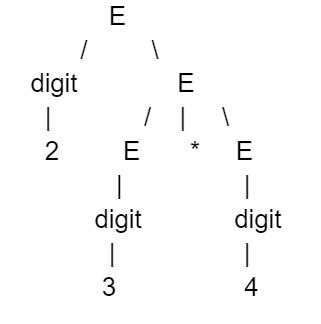In this parse tree:

• The root node represents the start symbol Expr.
• The left subtree represents the left operand of the addition operation, which is the digit 2.
• The right subtree represents the right operand of the addition operation, which is the expression (3 * 4).
• The right subtree has its own structure, with the left subtree representing the left operand of the multiplication operation (digit 3) and the right subtree representing the right operand (digit 4).

By following the tree from the root to the leaves, you can reconstruct the original expression “2 + (3 * 4)”.

Parse trees provide a visual representation of the syntactic structure of a string according to a given grammar. They can be used to verify the correctness of a parsing algorithm, analyze the structure of the parsed expression, and facilitate further processing or interpretation of the input.

There are different types of derivation trees based on the derivation process and the order of applying production rules. The main types are leftmost derivation trees, rightmost derivation trees, and ambiguous derivation trees. Let’s explore each type with examples:

1. Leftmost Derivation Tree:

A leftmost derivation tree is constructed by always expanding the leftmost non-terminal in each step of the derivation. It represents the leftmost derivation process.

1. Rightmost Derivation Tree:

A rightmost derivation tree is constructed by always expanding the rightmost non-terminal in each step of the derivation. It represents the rightmost derivation process.

Let’s consider the following grammar and string:

Grammar:

• Start symbol: S
• Production rules:
1. S → aSb
2. S → ab

String: “aaabbb”

Leftmost Derivation:

1. Leftmost derivation steps:
2. S → aSb (Applying rule 1)
3. S → aaSbb (Applying rule 1)
4. S → aaaSbbb (Applying rule 1)
5. S → aaabbb (Applying rule 2)

Leftmost derivation:

S → aSb → aaSbb → aaaSbbb → aaabbb

Leftmost derivation/parse tree: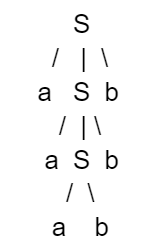Rightmost Derivation:

1. Rightmost derivation steps:
2. S → aSb (Applying rule 1)
3. S → aaSbb (Applying rule 1)
4. S → aaabbb (Applying rule 2)

Rightmost derivation:

S → aSb → aaSbb → aaabbb

Rightmost derivation/parse tree:Both the leftmost and rightmost derivation parse trees and derivations correctly demonstrate the derivation process for the given grammar and string “aaabbb”.

Example 1: Draw derivation tree for the given grammar and input string

1. E = E + E
2. E = E * E
3. E = a | b | c

Input string: a * b + c

Step 1: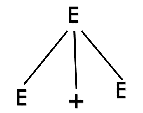Step 2: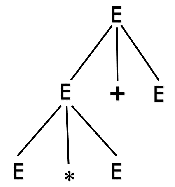Step 2: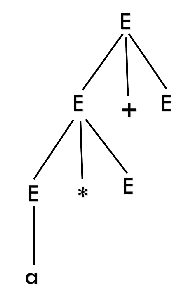Step 4: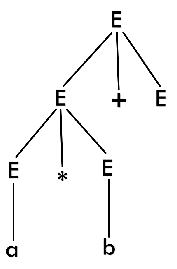Step 5: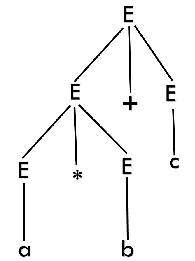Example 2: Draw a derivation tree for the string “bbabb” from the CFG given by

1. S → bSb | a | b

Solution: Now, the derivation tree for the string “bbabb” is as follows: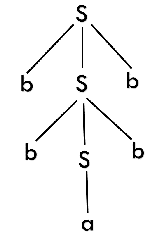The above tree is a derivation tree drawn for deriving a string bbabb. By simply reading the leaf nodes, we can obtain the desired string. The same tree can also be denoted by,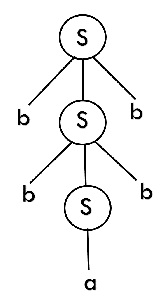Example 3: Construct a derivation tree for the string “aabbabba” for the CFG given by,

1. S → aB | bA
2. A → a | aS | bAA
3. B → b | bS | aBB

Solution: To draw a tree, we will first try to obtain derivation for the string aabbabba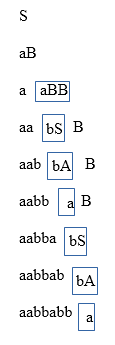Now, the derivation tree is as follows: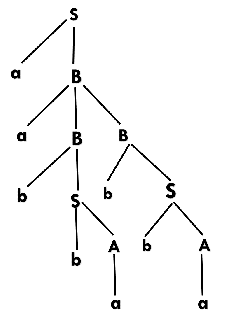Example 4: Show the derivation tree for string “aabbbb” with the following grammar.

1. S → AB | ε
2. A → aB
3. B → Sb

Solution: To draw a tree we will first try to obtain derivation for the string aabbbb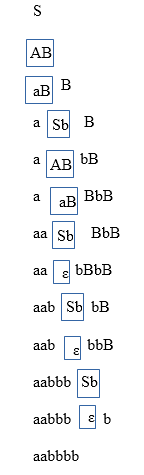Now, the derivation tree for the string “aabbbb” is as follows: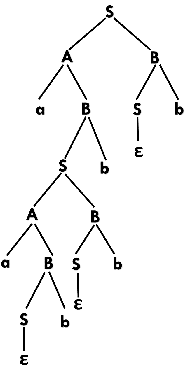Determine Ambiguity in CFG

To determine ambiguity in a context-free grammar (CFG), we need to check if there exist multiple parse trees for at least one string generated by the grammar. If multiple parse trees exist, it indicates ambiguity in the grammar.

Here’s a step-by-step approach to determine ambiguity in a CFG:

1. Identify a string generated by the grammar that you suspect might have multiple parse trees.
2. Construct the parse trees for that string using different derivation strategies, such as leftmost derivation and rightmost derivation.
3. If you obtain multiple distinct parse trees for the same string using different derivation strategies, it indicates ambiguity in the grammar.
4. Analyze the production rules and grammar structure to identify the source of ambiguity. Look for overlapping or conflicting production rules, or situations where the same string can be derived in multiple ways.
5. If you find that different parse trees lead to different interpretations or meanings for the same string, it further confirms ambiguity in the grammar.
6. It’s also essential to consider the language generated by the CFG. Some languages inherently have ambiguity, while others may have ambiguity due to specific grammar rules.

By following this process, you can determine if a CFG is ambiguous by identifying multiple parse trees for a particular string generated by the grammar.

Example 1: Check whether the given grammar G is ambiguous or not.

1. E → E + E
2. E → E – E
3. E → id

Solution: From the above grammar String “id + id – id” can be derived in 2 ways:

First Leftmost derivation

1. E → E + E
2. → id + E
3. → id + E – E
4. → id + id – E
5. → id + id- id

Second Leftmost derivation

1. E → E – E
2. → E + E – E
3. → id + E – E
4. → id + id – E
5. → id + id – id

Since there are two leftmost derivation for a single string “id + id – id”, the grammar G is ambiguous.

Example 2: Check whether the given grammar G is ambiguous or not.

1. S → aSb | SS
2. S → ε

Solution: For the string “aabb” the above grammar can generate two parse trees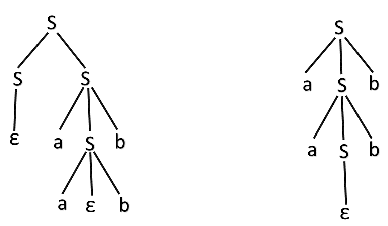Since there are two parse trees for a single string “aabb”, the grammar G is ambiguous.

Example 3: Check whether the given grammar G is ambiguous or not.

1. A → AA
2. A → (A)
3. A → a

Solution: For the string “a(a)aa” the above grammar can generate two parse trees: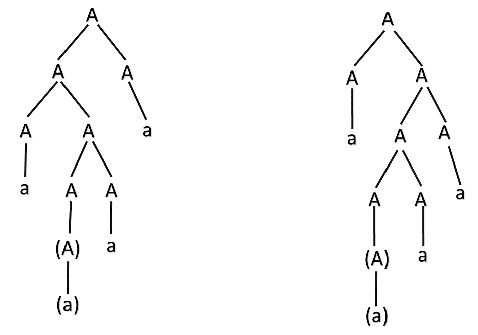Since there are two parse trees for a single string “a(a)aa”, the grammar G is ambiguous.

Converting Ambiguous Grammar Into Unambiguous Grammar:

To convert an ambiguous grammar into an unambiguous grammar, it is necessary to address causes such as left recursion and common prefixes, as they contribute to the ambiguity. By removing these causes, the grammar can be made unambiguous, although it is not always possible to do so.

There are several methods to remove ambiguity:

1. Fixing the grammar: By carefully redefining the production rules and eliminating left recursion or common prefixes, the grammar can be made unambiguous.
2. Adding grouping rules: By introducing additional rules to enforce grouping or precedence of certain expressions, ambiguity can be resolved.
3. Using semantics: By considering the meaning or context of the language being described by the grammar, it is possible to choose the parse that makes the most sense and eliminate ambiguity.
4. Adding precedence and associativity rules: By incorporating rules that define the precedence and associativity of operators, ambiguity can be resolved. Precedence rules establish the priority of operators based on the level of the production, while associativity rules determine whether an operator is left or right associative.

Implementing precedence and associativity constraints involves the following rules:

• R1: Precedence constraint: The level at which a production is defined determines the operator’s priority. Higher-level productions have lower priority, while lower-level productions have higher priority.
• R2: Associativity constraint: To enforce left associativity, induce left recursion in the production of the operator. To enforce right associativity, induce right recursion in the production.

By applying these techniques and rules, it is possible to convert an ambiguous grammar into an unambiguous one, improving clarity and eliminating multiple interpretations. However, it is important to note that complete conversion may not always be achievable for every ambiguous grammar.

Here are three examples that demonstrate the conversion of an ambiguous grammar to an unambiguous grammar:

Example 1: Ambiguous Grammar

Grammar:

• Start symbol: S
• Production rules:
1. S → aSb
2. S → SaaS
3. S → ε (epsilon)

To convert this grammar to an unambiguous grammar, we can modify it as follows:

Modified Unambiguous Grammar:

• Start symbol: S
• Production rules:
1. S → aSb
2. S → X
3. X → SaaS
4. X → ε (epsilon)

In this modified grammar, we introduce a new non-terminal symbol X to handle the ambiguity caused by the recursive rule S → SaaS. By separating the recursive part into a new production rule, we ensure that the grammar becomes unambiguous.

Example 2: Ambiguous Grammar

Grammar:

• Start symbol: E
• Production rules:
1. E → E + E
2. E → E * E
3. E → (E)
4. E → id

To convert this grammar to an unambiguous grammar, we can introduce precedence and associativity rules:

Modified Unambiguous Grammar:

• Start symbol: E
• Production rules:
1. E → E + T
2. E → T
3. T → T * F
4. T → F
5. F → (E)
6. F → id

In this modified grammar, we introduce non-terminal symbols T and F to represent different levels of precedence. The addition operation has a higher precedence than multiplication, ensuring unambiguous parsing.

Example 3: Ambiguous Grammar

Grammar:

• Start symbol: S
• Production rules:
1. S → aSa
2. S → bSb
3. S → ε (epsilon)

To convert this grammar to an unambiguous grammar, we can add grouping rules using parentheses:

Modified Unambiguous Grammar:

• Start symbol: S
• Production rules:
1. S → a(S)a
2. S → b(S)b
3. S → ε (epsilon)

In this modified grammar, we explicitly enforce the grouping of the terminals a and b by enclosing the non-terminal S in parentheses. This ensures unambiguous parsing by disallowing multiple interpretations of the input string.

These examples illustrate how to convert ambiguous grammars into unambiguous grammars by introducing new non-terminal symbols, precedence and associativity rules, or grouping rules. By addressing the causes of ambiguity and providing clear rules for parsing, the resulting unambiguous grammars eliminate any potential for multiple interpretations.

# Recall the procedure to Eliminate: Null Productions and Unit Productions

To eliminate null productions and unit productions from a context-free grammar, the following procedures can be applied:

1. Eliminating Null Productions:

Null productions are productions that derive the empty string (ε). To eliminate null productions, the following steps can be followed:

a. Identify the null productions in the grammar. These are the productions where ε appears on the right-hand side.
b. For each production that contains a null production, generate all possible combinations by removing the null production. This means creating new productions where the null production is omitted.
c. Repeat step b until no new null productions are generated.
d. Remove all the null productions from the grammar.

1. Eliminating Unit Productions:

Unit productions are productions where a single non-terminal symbol appears on the right-hand side. To eliminate unit productions, the following steps can be followed:

a. Identify the unit productions in the grammar.
b. For each unit production A → B, where A and B are non-terminal symbols, replace every occurrence of A with B in other productions.
c. Repeat step b until no new unit productions are generated.
d. Remove all the unit productions from the grammar.

After applying these procedures, the resulting grammar will have no null productions (productions deriving ε) or unit productions (productions with a single non-terminal symbol on the right-hand side). This process helps simplify the grammar and allows for more straightforward parsing and analysis.

It’s important to note that the elimination of null and unit productions may result in changes to the language generated by the grammar. Care should be taken to ensure that the transformed grammar still produces the desired language.

Here are two examples for each procedure: eliminating null productions and eliminating unit productions.

1. Examples of Eliminating Null Productions:

Example 1:

Grammar:

1. S → AB
2. A → ε
3. B → CD
4. C → ε
5. D → E

Procedure:

a. Identify the null productions: A → ε and C → ε.

b. Generate new productions without the null productions:

• S → AB | B
• A → ε
• B → CD
• C → ε
• D → E

c. Repeat step b:

• S → AB | B | CD | D | E
• A → ε
• B → CD | D | E
• C → ε
• D → E

d. Remove null productions:

• S → AB | B | CD | D | E
• B → CD | D | E
• D → E

The resulting grammar has eliminated the null productions.

Example 2:

Grammar:

1. S → A | B
2. A → ε
3. B → CD
4. C → ε
5. D → ε

Procedure:

a. Identify the null productions: A → ε, C → ε, and D → ε.

b. Generate new productions without the null productions:

• S → A | B
• B → CD

c. Repeat step b:

• S → A | B | CD
• B → CD

d. Remove null productions:

• S → A | B | CD
• B → CD

The resulting grammar has eliminated the null productions.

Example 1 to eliminate Unit productions.

Grammar:

1. S → A
2. A → B
3. B → a

Procedure:

a. Identify the unit productions: S → A, A → B.

b. Replace each unit production with the productions of the non-unit symbol:

• S → B
• A → a

c. Repeat step b until no new unit productions are generated.

The resulting grammar after eliminating unit productions:

1. S → a
2. A → a
3. B → a

In this example, the grammar initially contains the unit productions S → A and A → B. By replacing the unit productions with the productions of the non-unit symbols, we obtain the resulting grammar where each production drives a terminal symbol.

Example 2 to Eliminate unit productions.

Grammar:

1. S → A
2. A → B
3. B → C
4. C → D
5. D → a

Procedure:

a. Identify the unit productions: S → A, A → B, B → C, C → D.

b. Replace each unit production with the productions of the non-unit symbol:

• S → D
• A → D
• B → D
• C → a
• D → a

c. Repeat step b until no new unit productions are generated.

The resulting grammar after eliminating unit productions:

1. S → a
2. A → a
3. B → a
4. C → a
5. D → a

In this example, the grammar initially contains the unit productions S → A, A → B, B → C, and C → D. By replacing the unit productions with the productions of the non-unit symbols, we obtain the resulting grammar where each production drives a terminal symbol.

# Recall the procedure to find the Reduced Grammar for a given CFG

To find the reduced grammar for a given Context-Free Grammar (CFG), you can follow the following procedure:

1. Remove unreachable symbols: Start by identifying all the non-terminals and terminals that are not reachable from the start symbol. Remove any productions involving these symbols.
2. Remove useless symbols: Identify all the non-terminals and terminals that do not derive any string of terminals. Remove any productions involving these symbols.
3. Remove ε-productions: Identify any productions of the form A → ε, where A is a non-terminal. Remove these ε-productions.
4. Remove unit productions: Identify any unit productions of the form A → B, where both A and B are non-terminals. Replace each unit production with the productions of the non-unit symbol.
5. Eliminate left-recursion: If the grammar contains left-recursive productions, eliminate them using appropriate techniques such as left-recursion elimination or left-factoring.
6. Simplify the grammar: Perform any additional simplifications or transformations to make the grammar more concise and readable, if desired.

By following these steps, you can obtain the reduced grammar for a given CFG. Each step aims to eliminate certain types of productions or symbols to simplify and refine the grammar.

Please note that some steps may not be applicable to every grammar, and the specific techniques used for each step may vary depending on the requirements and constraints of the grammar.

Here are two examples to illustrate the procedure of finding the reduced grammar:

Example 1:

Consider the following grammar:

Grammar:

1. S → Aa
2. A → Aa | ε
3. B → b

Procedure:

1. Remove unreachable symbols: None in this example.
2. Remove useless symbols: None in this example.
3. Remove ε-productions: A → ε
4. Remove unit productions: None in this example.
5. Eliminate left-recursion: None in this example.

The resulting reduced grammar:

1. S → Aa
2. A → Aa
3. A → a
4. B → b

Example 2:

Consider the following grammar:

Grammar:

1. S → A | B | ε
2. A → aA | ε
3. B → bB | ε
4. C → cC

Procedure:

1. Remove unreachable symbols: None in this example.
2. Remove useless symbols: None in this example.
3. Remove ε-productions: S → ε, A → ε, B → ε
4. Remove unit productions: None in this example.
5. Eliminate left-recursion: None in this example.

The resulting reduced grammar:

1. S → A | B
2. A → aA | a
3. B → bB | b
4. C → cC

In both examples, we follow the procedure step-by-step to find the reduced grammar by eliminating unreachable symbols, useless symbols, ε-productions, and left-recursion (if applicable). The resulting reduced grammars are simpler and more concise, representing the same language as the original grammars.

# Define Chomsky Normal Form (CNF)

Chomsky Normal Form (CNF) is a standard form used to represent context-free grammars (CFGs). In CNF, every production rule in the grammar has one of two forms:

1. A → BC: Here, A, B, and C are non-terminal symbols, and BC represents a combination of two non-terminals.
2. A → a: Here, A is a non-terminal symbol, and a is a terminal symbol.

In other words, each production rule in CNF either produces two non-terminals or a single terminal. CNF has the following additional properties:

1. The start symbol of the grammar cannot appear on the right side of any production rule.
2. The empty string ε is not allowed in CNF, except for the case where the start symbol is allowed to derive ε.

The purpose of converting a CFG into Chomsky Normal Form is to simplify and analyze the grammar. CNF makes it easier to reason about the language generated by the grammar and enables more efficient parsing algorithms.

It is important to note that not all CFGs can be directly converted into CNF. Some grammars may require additional transformations or modifications before they can be represented in CNF.

# Recall the procedure to find CNF equivalent to the given CFG

To find the Chomsky Normal Form (CNF) equivalent of a given context-free grammar (CFG), you can follow the following procedure:

1. Remove ε-productions:
• Identify and remove any ε-productions (productions that derive the empty string).
• Adjust other productions accordingly by removing occurrences of the non-terminal symbol associated with the ε-production.
2. Remove unit productions:
• Identify and remove any unit productions (productions of the form A → B, where A and B are non-terminal symbols).
• Adjust other productions accordingly by replacing occurrences of the non-terminal symbol associated with the unit production.
3. Eliminate non-terminal symbols on the right side with more than two symbols:
• For each production with a right side containing more than two symbols, introduce new non-terminal symbols and break the production into smaller productions.
• Replace the original production with the new productions.
4. Convert non-terminal symbols on the right side into individual non-terminals:
• For each production with non-terminal symbols on the right side, replace them with new non-terminal symbols.
• Introduce new productions to represent the original relationships.
5. The resulting grammar is in CNF:
• All productions are either of the form A → BC (where A, B, and C are non-terminals) or A → a (where A is a non-terminal and a is a terminal).

It’s important to note that the above procedure assumes the given CFG is in a proper form without any syntax errors. Additionally, converting a CFG into CNF may result in an increase in the number of productions.

By following this procedure, you can obtain the CNF equivalent of a given CFG, which is useful for various applications such as parsing and analysis of context-free languages.

Example 1: Convert the given CFG to CNF. Consider the given grammar:

1. S → a | aA | B
2. A → aBB | ε
3. B → Aa | b

Solution:

Step 1: We will create a new production S1 → S, as the start symbol S appears on the RHS. The grammar will be:

1. S1 → S
2. S → a | aA | B
3. A → aBB | ε
4. B → Aa | b

Step 2: As grammar G1 contains A → ε null production, its removal from the grammar yields:

1. S1 → S
2. S → a | aA | B
3. A → aBB
4. B → Aa | b | a

Now, as grammar contains Unit production S → B, its removal yield:

1. S1 → S
2. S → a | aA | Aa | b
3. A → aBB
4. B → Aa | b | a

Also remove the unit production S1 → S, its removal from the grammar yields:

1. S0 → a | aA | Aa | b
2. S → a | aA | Aa | b
3. A → aBB
4. B → Aa | b | a

Step 3: In the production rule S0 → aA | Aa, S → aA | Aa, A → aBB and B → Aa, terminal a exists on RHS with non-terminals. So we will replace terminal a with X:

1. S0 → a | XA | AX | b
2. S → a | XA | AX | b
3. A → XBB
4. B → AX | b | a
5. X → a

Step 4: In the production rule A → XBB, RHS has more than two symbols, removing it from grammar yield:

1. S0 → a | XA | AX | b
2. S → a | XA | AX | b
3. A → RB
4. B → AX | b | a
5. X → a
6. R → XB

Hence, for the given grammar, this is the required CNF.

# Define Greibach Normal Form (GNF)

Greibach Normal Form (GNF) is a specific form of context-free grammars (CFGs) named after Sheila Greibach. In GNF, all productions have the form A → aα, where A is a non-terminal symbol, a is a terminal symbol, and α is a possibly empty string of non-terminals.

More formally, a CFG is in Greibach Normal Form if every production is of the following form:

A → aα

where A is a non-terminal symbol, a is a terminal symbol, and α is a possibly empty string of non-terminals.

In GNF, the right-hand side of each production starts with a terminal symbol, followed by zero or more non-terminal symbols. This form provides a restricted yet powerful representation of context-free languages.

1. Deterministic Parsing: GNF allows for deterministic parsing since there is only one non-terminal symbol at the beginning of each production.
2. Leftmost Derivation: GNF allows for efficient leftmost derivations, making it easier to analyze the structure of the language.

It’s worth noting that not all context-free languages can be represented in GNF. However, for those that can, GNF provides a concise and unambiguous representation of the language.

# Recall the procedure to find GNF equivalent to the given CFG

To find the Greibach Normal Form (GNF) equivalent of a given context-free grammar (CFG), you can follow the following procedure:

1. Remove ε-productions:
• Identify and remove any ε-productions (productions that derive the empty string).
• Adjust other productions accordingly by removing occurrences of the non-terminal symbol associated with the ε-production.
2. Remove unit productions:
• Identify and remove any unit productions (productions of the form A → B, where A and B are non-terminal symbols).
• Adjust other productions accordingly by replacing occurrences of the non-terminal symbol associated with the unit production.
3. Eliminate non-terminal symbols on the right side with more than one symbol:
• For each production with a right side containing more than one symbol, introduce new non-terminal symbols and break the production into smaller productions.
• Replace the original production with the new productions.
4. Convert non-terminal symbols on the right side into individual non-terminals:
• For each production with non-terminal symbols on the right side, replace them with new non-terminal symbols.
• Introduce new productions to represent the original relationships.
5. Ensure productions are in GNF form:
• Ensure that each production satisfies the GNF requirements, which state that the right side of each production consists of either a single terminal symbol or starts with a single terminal symbol followed by zero or more non-terminal symbols.
6. The resulting grammar is in GNF.

It’s important to note that the above procedure assumes the given CFG is in a proper form without any syntax errors. Additionally, converting a CFG into GNF may result in an increase in the number of productions.

By following this procedure, you can obtain the GNF equivalent of a given CFG, which provides a structured and unambiguous representation of the grammar and is useful for various parsing and analysis applications.

Example 1: Convert the following CFG into GNF.

1. S → XB | AA
2. A → a | SA
3. B → b
4. X → a

Solution:

As the given grammar G is already in CNF and there is no left recursion, so we can skip step 1 and step 2 and directly go to step 3.

The production rule A → SA is not in GNF, so we substitute S → XB | AA in the production rule A → SA as:

1. S → XB | AA
2. A → a | XBA | AAA
3. B → b
4. X → a

The production rule S → XB and B → XBA is not in GNF, so we substitute X → a in the production rule S → XB and B → XBA as:

1. S → aB | AA
2. A → a | aBA | AAA
3. B → b
4. X → a

Now we will remove left recursion (A → AAA), we get:

1. S → aB | AA
2. A → aC | aBAC
3. C → AAC | ε
4. B → b
5. X → a

Now we will remove null production C → ε, we get:

1. S → aB | AA
2. A → aC | aBAC | a | aBA
3. C → AAC | AA
4. B → b
5. X → a

The production rule S → AA is not in GNF, so we substitute A → aC | aBAC | a | aBA in production rule S → AA as:

1. S → aB | aCA | aBACA | aA | aBAA
2. A → aC | aBAC | a | aBA
3. C → AAC
4. C → aCA | aBACA | aA | aBAA
5. B → b
6. X → a

The production rule C → AAC is not in GNF, so we substitute A → aC | aBAC | a | aBA in production rule C → AAC as:

1. S → aB | aCA | aBACA | aA | aBAA
2. A → aC | aBAC | a | aBA
3. C → aCAC | aBACAC | aAC | aBAAC
4. C → aCA | aBACA | aA | aBAA
5. B → b
6. X → a

Hence, this is the GNF form for the grammar G.

# Recall Closure properties of CFLs such as Union, Concatenation, Closure etc.

Here are the closure properties of context-free languages (CFLs) along with examples for each:

1. Union:
• Property: The union of two CFLs is also a CFL.
• Example: Let’s consider two CFLs, L1 = {a^n b^n | n ≥ 0} and L2 = {a^n c^n | n ≥ 0}. Both languages contain strings with an equal number of ‘a’ followed by an equal number of ‘b’ or ‘c’. The union of L1 and L2, denoted as L1 ∪ L2, is a CFL that consists of strings in either language. For example, L1 ∪ L2 contains strings like “ab”, “aabb”, “ac”, “aacc”, etc.
2. Concatenation:
• Property: The concatenation of two CFLs is also a CFL.
• Example: Let’s consider two CFLs, L1 = {a^n b^n | n ≥ 0} and L2 = {c^m d^m | m ≥ 0}. The concatenation of L1 and L2, denoted as L1L2, is a CFL that consists of strings where an ‘a’ followed by a ‘b’ is concatenated with a ‘c’ followed by a ‘d’. For example, L1L2 contains strings like “abcd”, “aabbccdd”, “aabbbbccdddd”, etc.
3. Kleene Closure (Star):
• Property: The Kleene closure of a CFL is also a CFL.
• Example: Let’s consider a CFL, L = {a^n | n ≥ 0}. The Kleene closure of L, denoted as L*, is a CFL that consists of strings formed by concatenating zero or more strings from L. For example, L* contains strings like “a”, “aa”, “aaa”, “aaaa”, etc., as well as the empty string ε.
4. Intersection:
• Property: The intersection of two CFLs is not necessarily a CFL.
• Example: Let’s consider two CFLs, L1 = {a^n b^n | n ≥ 0} and L2 = {a^n c^n | n ≥ 0}. The intersection of L1 and L2, denoted as L1 ∩ L2, is not a CFL. The language L1 ∩ L2 contains strings that are both in L1 and L2, which violates the closure property for intersection.
5. Complement:
• Property: The complement of a CFL is not necessarily a CFL.
• Example: Let’s consider a CFL, L = {a^n b^n | n ≥ 0}. The complement of L, denoted as L’, is not a CFL. The language L’ contains strings that are not in L, which includes strings with an unequal number of ‘a’s and ‘b’s, as well as strings that do not contain ‘a’ or ‘b’.

It’s important to note that while union, concatenation, and Kleene closure preserve the context-free property, intersection and complement do not necessarily result in a CFL.

# Recall decision properties of CFLs

Here are some decision properties of context-free languages (CFLs) along with examples for each:

1. Emptiness:
• Property: Determines whether a CFL is empty (contains no strings).
• Example: Consider a CFL L = {a^n b^n | n ≥ 0}. The emptiness problem for L asks whether L contains any strings. In this case, L is not empty because it contains strings like “ab”, “aabb”, “aaabbb”, etc.
2. Membership:
• Property: Determines whether a given string belongs to a CFL.
• Example: Consider a CFL L = {a^n b^n | n ≥ 0}. The membership problem for L asks whether a given string, such as “aabb”, belongs to L. In this case, “aabb” belongs to L because it follows the pattern of having an equal number of ‘a’s and ‘b’s.
3. Equality:
• Property: Determines whether two CFLs are equal, i.e., contain exactly the same set of strings.
• Example: Consider two CFLs, L1 = {a^n b^n | n ≥ 0} and L2 = {w | w is a palindrome}. The equality problem for L1 and L2 asks whether L1 and L2 are the same language. In this case, L1 and L2 are not equal because they contain different sets of strings. L1 only contains strings with equal numbers of ‘a’s and ‘b’s, while L2 contains strings that are palindromes.
4. Universality:
• Property: Determines whether a CFL contains all possible strings over its alphabet.
• Example: Consider a CFL L = {a^n b^n | n ≥ 0}. The universality problem for L asks whether L contains all possible strings over the alphabet {a, b}. In this case, L is not universal because it only contains strings with an equal number of ‘a’s and ‘b’s.
5. Intersection with Regular Language:
• Property: Determines whether the intersection of a CFL and a regular language is non-empty.
• Example: Consider a CFL L = {a^n b^n | n ≥ 0} and a regular language R = (ab)*. The intersection problem for L and R asks whether the intersection of L and R is non-empty. In this case, the intersection of L and R is non-empty because both languages contain the string “ab”.

These decision properties are used to analyze and classify context-free languages based on their properties and relationships with other languages.

# Recall CYK (Cocke-Younger-Kasami) Algorithm

The CYK (Cocke-Younger-Kasami) algorithm is a parsing algorithm used to determine if a given string can be generated by a given context-free grammar (CFG). The algorithm is based on dynamic programming and operates in a bottom-up manner.

Here’s an overview of the CYK algorithm:

1. Input:
• A CFG in Chomsky Normal Form (CNF) consisting of a set of non-terminal symbols, a set of terminal symbols, a set of production rules, and a start symbol.
• A string to be parsed.
2. Initialization:
• Create an n x n matrix, where n is the length of the input string.
• Initialize all cells of the matrix as empty.
3. Filling the matrix:
• For each cell (i, j) in the matrix, where i represents the starting position and j represents the ending position:
• If the substring from position i to j (inclusive) is a terminal symbol, populate the cell with non-terminals that produce that terminal.
• For each value of k from i to j-1:
• For each production rule A -> BC, where B and C are non-terminals:
• If the cell (i, k) contains non-terminal B and the cell (k+1, j) contains non-terminal C, populate the cell (i, j) with non-terminal A.
4. Parsing result:
• If the start symbol of the grammar is present in the cell (0, n-1), where n is the length of the input string, then the string can be generated by the grammar. Otherwise, it cannot.

The CYK algorithm uses the concept of subproblems and overlapping subproblems to efficiently determine the parseability of a string. By filling the matrix from smaller substrings to larger substrings, it systematically checks all possible ways to derive the string from the grammar. If the start symbol is present in the final cell of the matrix, it indicates that the string can be generated by the grammar.

The CYK algorithm has a time complexity of O(n^3 * |G|), where n is the length of the input string and |G| is the size of the CFG. It is widely used in natural language processing and syntax analysis to determine the grammaticality of sentences.

# Apply CYK Algorithm

The CYK algorithm can be used to decide the membership of a given string in a context-free grammar (CFG). The algorithm constructs a triangular table where each row represents a specific length of substrings. The bottommost row corresponds to substrings of length 1, the second row from the bottom corresponds to substrings of length 2, and so on. The topmost row represents the given string itself, which has a length of n. By populating the table with non-terminals that can generate the corresponding substrings, we can determine if the given string can be derived from the CFG.

Example: For a given string “x” of length 4 units, triangular table looks like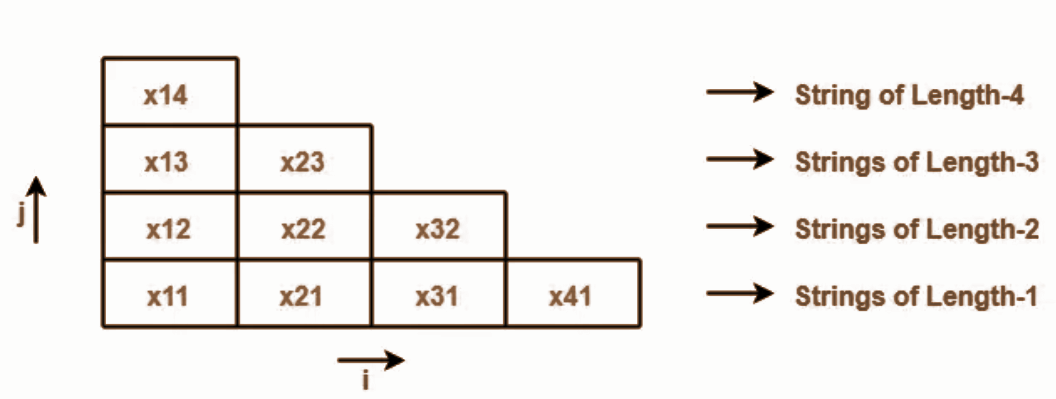We will fill each box of xij with its Vij. These notations are discussed below.

Where,

xij represents a sub string of “x” starting from location ‘i’ and has length ‘j’.

Example-

Consider x = abcd is a string, then

Number of sub strings possible = n(n+1)/2 = 4 x (4+1) / 2 = 10

We have-

• x11 = a
• x21 = b
• x31 = c
• x41 = d
• x12 = ab
• x22 = bc
• x32 = cd
• x13 = abc
• x23 = bcd
• x14 = abcd

andj

Vij represents a set of variables in the grammar which can derive the sub string xij.

If the set of variables consists of the start symbol, then it becomes sure-

• Sub-string xij can be derived from the given grammar.
• Sub-string xij is a member of the language of the given grammar.

Example: For the given grammar, check the acceptance of string w = baaba using CYK Algorithm.

S → AB / BC

A → BA / a

B → CC / b

C → AB / a

Explanation:

First, let us draw the triangular table.

• The given string is x = baaba.
• Length of given string = |x| = 5.
• So, Number of sub strings possible = (5 x 6) / 2 = 15.

So, triangular table looks like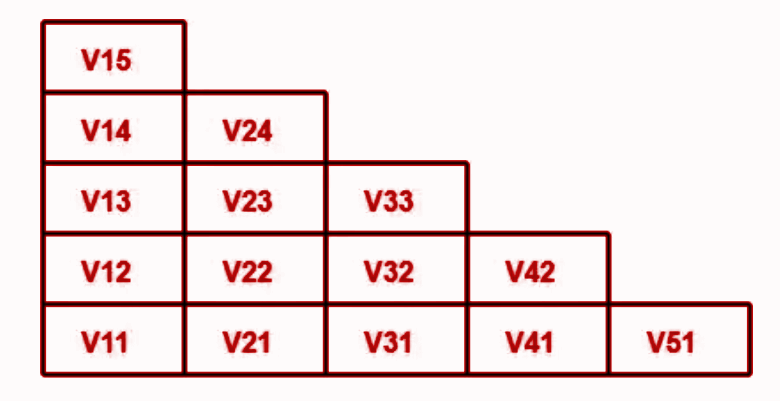Now, let us find the value of Vij for each cell.

For first row:

Evaluate V11

• V11 represents the set of variables deriving x11.
• x11 = b.
• Only variable B derives string “b” in the given grammar.
• Thus, V11 = { B }

Evaluate V21

• V21 represents the set of variables deriving x21.
• x21 = a.
• Variables A and C derive string “a” in the given grammar.
• Thus, V21 = { A , C }

Evaluate V31

• V31 represents the set of variables deriving x31.
• x31 = a.
• Variables A and C derive string “b” in the given grammar.
• Thus, V31 = { A , C }

Evaluate V41

• V41 represents the set of variables deriving x41.
• x41 = b.
• Only variable B derives string “b” in the given grammar.
• Thus, V41 = { B }

Evaluate V51

• V51 represents the set of variables deriving x51.
• x51 = a.
• Variables A and C derives string “a” in the given grammar.
• Thus, V51 = { A , C }

For Second row

As per the algorithm, to find the value of Vij from 2nd row on wards,

we use the formula-

Vij = Vik V(i+k)(j-k)

where k varies from 1 to j-1

Evaluate V12

We have i = 1 , j = 2 , k = 1

Substituting values in the formula, we get-

V12 = V11. V21

V12 = { B } { A , C }

V12 = { BA , BC }

∴ V12 = { A , S }

Evaluate V22

We have i = 2 , j = 2 , k = 1

Substituting values in the formula, we get-

V22 = V21. V31

V22 = { A , C } { A , C }

V22 = { AA , AC , CA , CC }

Since AA , AC and CA do not exist, so we have-

V22 = { CC }

∴ V22 = { B }

Evaluate V32

We have i = 3 , j = 2 , k = 1

Substituting values in the formula, we get-

V32 = V31. V41

V32 = { A , C } { B }

V32 = { AB , CB }

Since CB does not exist, so we have-

V32 = { AB }

∴ V32 = { S , C }

Evaluate V42

We have i = 4 , j = 2 , k = 1

Substituting values in the formula, we get-

V42 = V41. V51

V42 = { B } { A , C }

V42 = { BA , BC }

∴ V42 = { A , S }

For third row:

Evaluate V13

We have i = 1 , j = 3 , k = 1 to (3-1) = 1,2

Substituting values in the formula, we get-

V13 = V11. V22 ∪ V12. V31

V13 = { B } { B } ∪ { A , S } { A , C }

V13 = { BB } ∪ { AA , AC , SA , SC }

Since BB , AA , AC , SA and SC do not exist, so we have-

V13 = ϕ ∪ ϕ

∴ V13 = ϕ

Evaluate V23

We have i = 2 , j = 3 , k = 1 to (3-1) = 1,2

Substituting values in the formula, we get-

V23 = V21. V32 ∪ V22. V41

V23 = { A , C } { S , C } ∪ { B } { B }

V23 = { AS , AC , CS , CC } ∪ { BB }

Since AS , AC , CS and BB do not exist, so we have-

V23 = { CC }

∴ V23 = B

Evaluate V33

We have i = 3 , j = 3 , k = 1 to (3-1) = 1,2

Substituting values in the formula, we get-

V33 = V31. V42 ∪ V32. V51

V33 = { A , C } { A , S } ∪ { S , C } { A , C }

V33 = { AA , AS , CA , CS } ∪ { SA , SC , CA , CC }

Since AA , AS , CA , CS , SA , SC and CA do not exist, so we have-

V33 = ϕ ∪ { CC }

V33 = ϕ ∪ { B }

∴ V33 = { B }

For fourth row onward:

Evaluate V14

We have i = 1 , j = 4 , k = 1 to (4-1) = 1,2,3

Substituting values in the formula, we get-

V14 = V11. V23 ∪ V12. V32 ∪ V13. V41

V14 = { B } { B } ∪ { A , S } { S , C } ∪ { ϕ , B }

V14 = { BB } ∪ { AS , AC , SS , SC } ∪ { B }

Since BB , AS , AC , SS , SC and B do not exist, so we have-

V14 = ϕ ∪ ϕ ∪ ϕ

∴ V14 = ϕ

Evaluate V24

We have i = 2 , j = 4 , k = 1 to (4-1) = 1,2,3

Substituting values in the formula, we get-

V24 = V21. V33 ∪ V22. V42 ∪ V23. V51

V24 = { A , C } { B } ∪ { B } { A , S } ∪ { B } { A , C }

V24 = { AB , CB } ∪ { BA , BS } ∪ { BA , BC }

Since CB does not exist, so we have-

V24 = { AB } ∪ { BA , BS } ∪ { BA , BC }

V24 = { S , C } ∪ { A } ∪ { A , S }

∴ V24 = { S , C , A }

For fifth row:

Evaluate V15

We have i = 1 , j = 5 , k = 1 to (5-1) = 1,2,3,4

Substituting values in the formula, we get-

V15 = V11. V24 ∪ V12. V33 ∪ V13. V42 ∪ V14. V51

V15 = { B } { S , C , A } ∪ { A , S } { B } ∪ { ϕ } { A , S } ∪ { ϕ } { A , C }

V15 = { BS , BC , BA } ∪ { AB , SB } ∪ { A , S } ∪ { A , C }

Since BS , SB , A , S and C do not exist, so we have-

V15 = { BC , BA } ∪ { AB } ∪ ϕ ∪ ϕ

V15 = { S , A } ∪ { S , C } ∪ ϕ ∪ ϕ

∴ V15 = { S , A , C }

Now,

• The value of Vij is computed for each cell.
• We observe V15 contains the start symbol S.
• Thus, string x15 = baaba is a member of the language of given grammar.

After filling the triangular table, it looks like-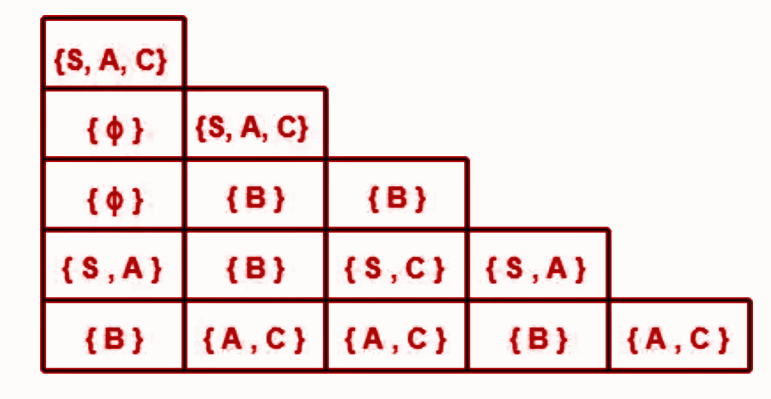Takeaway from the above triangular table:

Takeaway 1:

• There exists total 4 distinct sub strings which are members of the language of given grammar.
• These 4 sub strings are ba, ab, aaba, baaba.
• This is because they contain start symbol in their respective cell.

Takeaway 2:

• Strings which can not be derived from any variable are baa, baab.
• This is because they contain ϕ in their respective cell.

Takeaway 3:

• Strings which can be derived from variable B alone are b, aa, aba, aab.
• This is because they contain variable B alone in their respective cell.

# Recall Pumping Lemma for CFLs

The Pumping Lemma for context-free languages (CFLs) is a property that can be used to prove that a language is not context-free. It states that for every context-free language L, there exists a pumping length p such that any string in L of length at least p can be divided into five parts, uvxyz, satisfying the following conditions:

1. For each non-negative integer i, the string u(v^i)x(y^i)z is also in L.
2. The length of the substring vy is greater than 0.
3. The length of the string u(v^i)x(y^i)z is less than or equal to p.

In simpler terms, the Pumping Lemma states that if a language L is context-free, then there exists a length p such that any sufficiently long string in L can be “pumped” by repeating a portion of the string any number of times while still remaining in L.

The Pumping Lemma is a useful tool for proving that certain languages are not context-free. If we can show that the conditions of the Pumping Lemma cannot be satisfied for a given language, then we can conclude that the language is not context-free.

It’s important to note that the Pumping Lemma only provides a necessary condition for a language to be context-free. There are context-free languages that do not satisfy the conditions of the Pumping Lemma, and there are non-context-free languages that do satisfy the conditions. Therefore, the Pumping Lemma cannot be used to prove that a language is context-free, but it can be used to identify languages that are not context-free.

# Apply Pumping Lemma for CFLs

Let’s apply the Pumping Lemma for CFLs to a specific language and demonstrate that it is not context-free.

Example 1: Consider the language L = {a^n b^n c^n | n >= 0}, which consists of strings containing an equal number of ‘a’s, ‘b’s, and ‘c’s, arranged in the same order. We will show that L is not a context-free language using the Pumping Lemma.

Assume that L is a context-free language. Let p be the pumping length given by the Pumping Lemma.

1. Choose a string w = a^p b^p c^p from L. The length of w is p, which satisfies the condition of the Pumping Lemma.
2. According to the Pumping Lemma, we can divide w into five parts: uvxyz, where |vxy| <= p and |vy| > 0. Let’s consider the possible placements of vxy within w.
a) vxy contains only ‘a’s: In this case, pumping up or down will result in an unequal number of ‘a’s, ‘b’s, and ‘c’s, violating the condition of L.
b) vxy contains only ‘b’s: Pumping up or down will result in an unequal number of ‘a’s, ‘b’s, and ‘c’s, violating the condition of L.
c) vxy contains only ‘c’s: Pumping up or down will result in an unequal number of ‘a’s, ‘b’s, and ‘c’s, violating the condition of L.
d) vxy contains both ‘a’s and ‘b’s: Pumping up will introduce an unequal number of ‘a’s and ‘b’s, violating the condition of L.
e) vxy contains both ‘b’s and ‘c’s: Pumping up will introduce an unequal number of ‘b’s and ‘c’s, violating the condition of L.
f) vxy contains both ‘a’s and ‘c’s: Pumping up will introduce an unequal number of ‘a’s and ‘c’s, violating the condition of L.
g) vxy contains ‘a’s, ‘b’s, and ‘c’s: Pumping up will result in an unequal number of ‘a’s, ‘b’s, and ‘c’s, violating the condition of L.
3. In all possible cases, pumping the string w violates the condition of L. This contradicts the assumption that L is a context-free language.

Therefore, we have shown that the language L = {a^n b^n c^n | n >= 0} is not a context-free language using the Pumping Lemma.

Example 2: L = {ww^R | w is a string of 0s and 1s}

Assume L is a context-free language and let p be the pumping length given by the Pumping Lemma.

1. Choose a string w = 0^p 1^p 0^p from L. The length of w is 3p, which satisfies the condition of the Pumping Lemma.
2. Divide w into five parts: uvxyz, where |vxy| <= p and |vy| > 0. Consider the possible placements of vxy within w.
a) vxy contains only 0s: Pumping up or down will result in an unequal number of 0s in the two halves of the string, violating the condition of L.
b) vxy contains only 1s: Pumping up or down will result in an unequal number of 1s in the two halves of the string, violating the condition of L.
c) vxy contains both 0s and 1s: Pumping up will result in an unequal number of 0s and 1s in the two halves of the string, violating the condition of L.
3. In all possible cases, pumping the string w violates the condition of L. Therefore, L = {ww^R | w is a string of 0s and 1s} is not a context-free language.

Example 3: L = {a^n b^n c^n d^n | n >= 0}

Assume L is a context-free language and let p be the pumping length given by the Pumping Lemma.

1. Choose a string w = a^p b^p c^p d^p from L. The length of w is 4p, which satisfies the condition of the Pumping Lemma.
2. Divide w into five parts: uvxyz, where |vxy| <= p and |vy| > 0. Consider the possible placements of vxy within w.
a) vxy contains only ‘a’s: Pumping up or down will result in an unequal number of ‘a’s, ‘b’s, ‘c’s, and ‘d’s, violating the condition of L.
b) vxy contains only ‘b’s: Pumping up or down will result in an unequal number of ‘b’s, ‘c’s, and ‘d’s, violating the condition of L.
c) vxy contains only ‘c’s: Pumping up or down will result in an unequal number of ‘c’s and ‘d’s, violating the condition of L.
d) vxy contains only ‘d’s: Pumping up or down will result in an unequal number of ‘d’s, violating the condition of L.
e) vxy contains both ‘a’s and ‘b’s: Pumping up will introduce an unequal number of ‘a’s and ‘b’s, violating the condition of L.
f) vxy contains both ‘b’s and ‘c’s: Pumping up will introduce an unequal number of ‘b’s and ‘c’s, violating the condition of L.
g) vxy contains both ‘c’s and ‘d’s: Pumping up will introduce an unequal number of ‘c’s and ‘d’s, violating the condition of L.
h) vxy contains both ‘a’s and ‘c’s: Pumping up will introduce an unequal number of ‘a’s and ‘c’s, violating the condition of L.
i) vxy contains both ‘b’s and ‘d’s: Pumping up will introduce an unequal number of ‘b’s and ‘d’s, violating the condition of L.
3. In all possible cases, pumping the string w violates the condition of L. Therefore, L = {a^n b^n c^n d^n | n >= 0} is not a context-free language.

Example 4: L = {a^i b^j c^k | i < j < k}

Assume L is a context-free language and let p be the pumping length given by the Pumping Lemma.

1. Choose a string w = a^p b^(p+1) c^(p+2) from L. The length of w is 3p + 3, which satisfies the condition of the Pumping Lemma.
2. Divide w into five parts: uvxyz, where |vxy| <= p and |vy| > 0. Consider the possible placements of vxy within w.
Since the condition i < j < k holds for strings in L, it is not possible to choose vxy in a way that satisfies the conditions of the Pumping Lemma. The difference in the number of ‘a’s, ‘b’s, and ‘c’s cannot be maintained when pumping up or down.
3. The inability to satisfy the conditions of the Pumping Lemma indicates that L = {a^i b^j c^k | i < j < k} is not a context-free language.

Therefore, in all the given examples, the languages are not context-free as they violate the conditions of the Pumping Lemma.

Example 5: L = {a^n b^n c^n d^m | n, m >= 0}

Assume L is a context-free language and let p be the pumping length given by the Pumping Lemma.

1. Choose a string w = a^p b^p c^p d^p from L. The length of w is 4p, which satisfies the condition of the Pumping Lemma.
2. Divide w into five parts: uvxyz, where |vxy| <= p and |vy| > 0. Consider the possible placements of vxy within w.
a) vxy contains only ‘a’s: Pumping up or down will result in an unequal number of ‘a’s, violating the condition of L.
b) vxy contains only ‘b’s: Pumping up or down will result in an unequal number of ‘b’s, violating the condition of L.
c) vxy contains only ‘c’s: Pumping up or down will result in an unequal number of ‘c’s, violating the condition of L.
d) vxy contains only ‘d’s: Pumping up or down will result in an unequal number of ‘d’s, violating the condition of L.
e) vxy contains both ‘a’s and ‘b’s: Pumping up or down will introduce an unequal number of ‘a’s and ‘b’s, violating the condition of L.
f) vxy contains both ‘b’s and ‘c’s: Pumping up or down will introduce an unequal number of ‘b’s and ‘c’s, violating the condition of L.
g) vxy contains both ‘c’s and ‘d’s: Pumping up or down will introduce an unequal number of ‘c’s and ‘d’s, violating the condition of L.
h) vxy contains both ‘a’s and ‘c’s: Pumping up or down will introduce an unequal number of ‘a’s and ‘c’s, violating the condition of L.
i) vxy contains both ‘b’s and ‘d’s: Pumping up or down will introduce an unequal number of ‘b’s and ‘d’s, violating the condition of L.
j) vxy contains both ‘a’s and ‘d’s: Pumping up or down will introduce an unequal number of ‘a’s and ‘d’s, violating the condition of L.
k) vxy contains both ‘c’s and ‘d’s: Pumping up or down will introduce an unequal number of ‘c’s and ‘d’s, violating the condition of L.
l) vxy contains ‘a’s, ‘b’s, and ‘c’s: Pumping up or down will result in an unequal number of ‘a’s, ‘b’s, and ‘c’s, violating the condition of L.
m) vxy contains ‘b’s, ‘c’s, and ‘d’s: Pumping up or down will result in an unequal number of ‘b’s, ‘c’s, and ‘d’s, violating the condition of L.
n) vxy contains ‘a’s, ‘c’s, and ‘d’s: Pumping up or down will result in an unequal number of ‘a’s, ‘c’s, and ‘d’s, violating the condition of L.
o) vxy contains ‘a’s, ‘b’s, and ‘d’s: Pumping up or down will result in an unequal number of ‘a’s, ‘b’s, and ‘d’s, violating the condition of L.
p) vxy contains ‘a’s, ‘b’s, ‘c’s, and ‘d’s: Pumping up or down will result in an unequal number of ‘a’s, ‘b’s, ‘c’s, and ‘d’s, violating the condition of L.
3. In all possible cases, pumping the string w violates the condition of L. Therefore, L = {a^n b^n c^n d^m | n, m >= 0} is not a context-free language.

Example 6: L = {a^n b^m c^m d^n | n, m >= 0}

Assume L is a context-free language and let p be the pumping length given by the Pumping Lemma.

1. Choose a string w = a^p b^p c^p d^p from L. The length of w is 4p, which satisfies the condition of the Pumping Lemma.
2. Divide w into five parts: uvxyz, where |vxy| <= p and |vy| > 0. Consider the possible placements of vxy within w.
a) vxy contains only ‘a’s: Pumping up or down will result in an unequal number of ‘a’s, violating the condition of L.
b) vxy contains only ‘b’s: Pumping up or down will result in an unequal number of ‘b’s, violating the condition of L.
c) vxy contains only ‘c’s: Pumping up or down will result in an unequal number of ‘c’s, violating the condition of L.
d) vxy contains only ‘d’s: Pumping up or down will result in an unequal number of ‘d’s, violating the condition of L.
e) vxy contains both ‘a’s and ‘b’s: Pumping up or down will introduce an unequal number of ‘a’s and ‘b’s, violating the condition of L.
f) vxy contains both ‘b’s and ‘c’s: Pumping up or down will introduce an unequal number of ‘b’s and ‘c’s, violating the condition of L.
g) vxy contains both ‘c’s and ‘d’s: Pumping up or down will introduce an unequal number of ‘c’s and ‘d’s, violating the condition of L.
h) vxy contains both ‘a’s and ‘c’s: Pumping up or down will introduce an unequal number of ‘a’s and ‘c’s, violating the condition of L.
i) vxy contains both ‘b’s and ‘d’s: Pumping up or down will introduce an unequal number of ‘b’s and ‘d’s, violating the condition of L.
j) vxy contains both ‘a’s and ‘d’s: Pumping up or down will introduce an unequal number of ‘a’s and ‘d’s, violating the condition of L.
k) vxy contains both ‘c’s and ‘d’s: Pumping up or down will introduce an unequal number of ‘c’s and ‘d’s, violating the condition of L.
l) vxy contains ‘a’s, ‘b’s, and ‘c’s: Pumping up or down will result in an unequal number of ‘a’s, ‘b’s, and ‘c’s, violating the condition of L.
m) vxy contains ‘b’s, ‘c’s, and ‘d’s: Pumping up or down will result in an unequal number of ‘b’s, ‘c’s, and ‘d’s, violating the condition of L.
n) vxy contains ‘a’s, ‘c’s, and ‘d’s: Pumping up or down will result in an unequal number of ‘a’s, ‘c’s, and ‘d’s, violating the condition of L.
o) vxy contains ‘a’s, ‘b’s, and ‘d’s: Pumping up or down will result in an unequal number of ‘a’s, ‘b’s, and ‘d’s, violating the condition of L.
p) vxy contains ‘a’s, ‘b’s, ‘c’s, and ‘d’s: Pumping up or down will result in an unequal number of ‘a’s, ‘b’s, ‘c’s, and ‘d’s, violating the condition of L.
3. In all possible cases, pumping the string w violates the condition of L. Therefore, L = {a^n b^m c^m d^n | n, m >= 0} is not a context-free language.

Example 7: L= {a^p | p is Prime number}

To apply the Pumping Lemma for Context-Free Languages (CFLs) on the language L = {a^p | p is prime}, we need to show that for any string w in L that is sufficiently long, it can be divided into five parts uvxyz such that the following conditions hold:

1. |vxy| ≤ p: The length of vxy should be less than or equal to p.
2. |vy| > 0: The length of vy should be greater than zero.
3. For all k ≥ 0, the string u(v^k)x(y^k)z should also be in L.

Let’s assume that L is a CFL and let p be the pumping length given by the Pumping Lemma.

1. Choose a string w = a^p from L. The length of w is p, which satisfies the condition of the Pumping Lemma.
2. Divide w into five parts: uvxyz, where |vxy| ≤ p and |vy| > 0. Consider the possible placements of vxy within w.
a) vxy contains only ‘a’s: In this case, pumping up or down by increasing or decreasing the number of ‘a’s will result in a string that is not in L. Since p is a prime number, it cannot be divided into smaller factors, and pumping will generate a non-prime number of ‘a’s.
b) vxy contains both ‘a’s and other characters: Since w consists only of ‘a’s, any substring vxy containing other characters will violate the condition that all characters in w are ‘a’s.
c) vxy contains only other characters: In this case, pumping up or down will introduce additional characters other than ‘a’, resulting in a string that is not in L.
Since none of the possible placements of vxy allow for pumping while maintaining the property of having a prime number of ‘a’s, we can conclude that L = {a^p | p is prime} does not satisfy the conditions of the Pumping Lemma for CFLs.

Therefore, L = {a^p | p is prime} is not a context-free language.